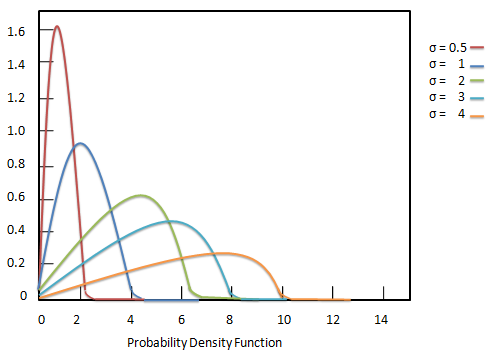# Statistics - Rayleigh Distribution

The Rayleigh distribution is a distribution of continuous probability density function. It is named after the English Lord Rayleigh. This distribution is widely used for the following:

• Communications - to model multiple paths of densely scattered signals while reaching a receiver.

• Physical Sciences - to model wind speed, wave heights, sound or light radiation.

• Engineering - to check the lifetime of an object depending upon its age.

• Medical Imaging - to model noise variance in magnetic resonance imaging.The probability density function Rayleigh distribution is defined as:

## Formula

${ f(x; \sigma) = \frac{x}{\sigma^2} e^{\frac{-x^2}{2\sigma^2}}, x \ge 0 }$

Where −

• ${\sigma}$ = scale parameter of the distribution.

The comulative distribution function Rayleigh distribution is defined as:

## Formula

${ F(x; \sigma) = 1 - e^{\frac{-x^2}{2\sigma^2}}, x \in [0 \infty}$

Where −

• ${\sigma}$ = scale parameter of the distribution.

## Variance and Expected Value

The expected value or the mean of a Rayleigh distribution is given by:

${ E[x] = \sigma \sqrt{\frac{\pi}{2}} }$

The variance of a Rayleigh distribution is given by:

${ Var[x] = \sigma^2 \frac{4-\pi}{2} }$